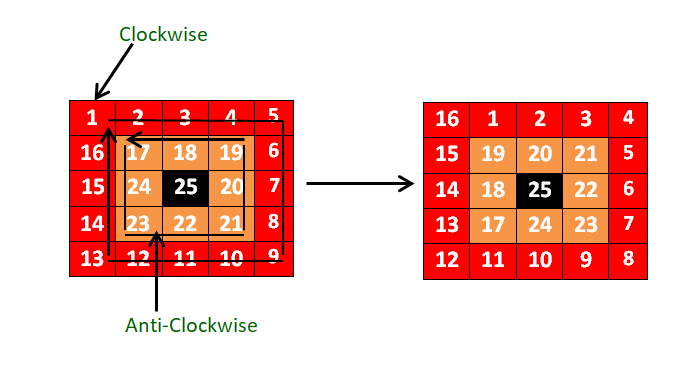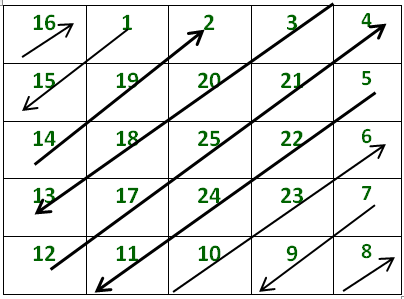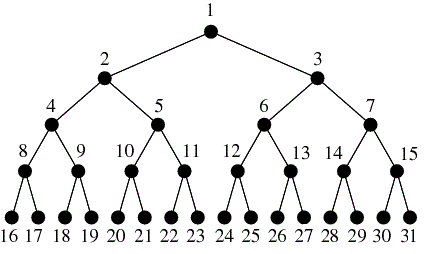Open In App

# Factset Interview Experience | Set 12 (On-Campus)

First Round(Pen and Paper based coding round)- 60 min

1. You are given an NxN matrix. You have to rotate same colored rectangle clockwise or anticlockwise alternatively by a ‘k’ value. K value keeps on increasing by one as we move from outer to the inner loop. Suppose in the given figure outermost loop is rotated by 1 place clockwise followed by next loop rotated by 2 places anticlockwise and so on. [Give only Inplace solution].2. You are given a binary tree. You have to calculate the sum of all nodes of a given tree without using recursion and extra space(e.g. no Queue/Stack).
3. You are given time in 24-hour format. You have to print the next palindromic time.
Example: 11:53 Output: 1221

24 students out of 170 were selected after coding round.

Technical Interview – I (35 min Approx.)
1. When you access any website through a web browser, it automatically converts web content to default language to that country to which the IP address of URL belongs. Use an efficient data structure to implement the above process. You have given a country name, language and IP range of that country.
2. A string is given in encoded form(e.g. [2a[2b[2c]]] ) You have to decode given string (O/P – abccbccabccbcc ).

3. A NxN matrix is given .You have to print matrix in given zigzag format.Technical Interview – II ( 1 hr 15 min approx.)
1. i) Do you have any idea about justification in MS word?? . Give the logic of how MS word implements Center justification. Write code for the logic that you have given.
ii) You have to implement center justification such that all left and right words of each row (line) are aligned and lie on the same column. You have to adjust extra spaces between the words. Give logic and also write the code. He also gave some test cases to run.

2. Give all approaches to find the majority element in the array. (From naïve to Moore voting algorithm approach)
3. i) You have to find a path from one leaf node to another leaf node which has a maximum sum in a given binary tree(Note: Without using recursion and extra space).

ii)Also, you have to print that path which has maximum sum without using stack/Queue in O(1) space. (Note: You can change the structure of a node ).

4. You are given a perfect binary tree. You have to print the kth level form the leaf node without calculating the height of the tree.5. A brief discussion on projects
8 students were selected for the HR interview.

HR Interview ( 20 min ) –